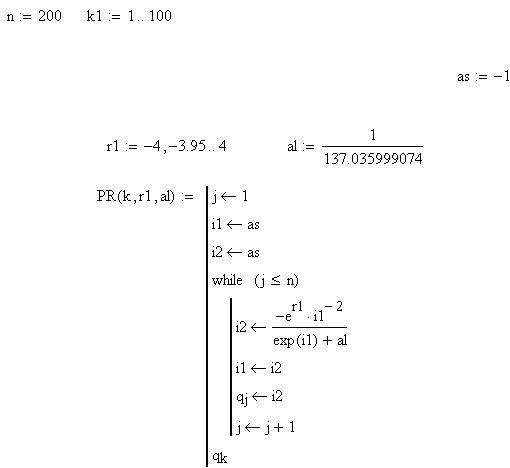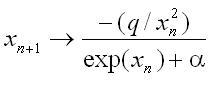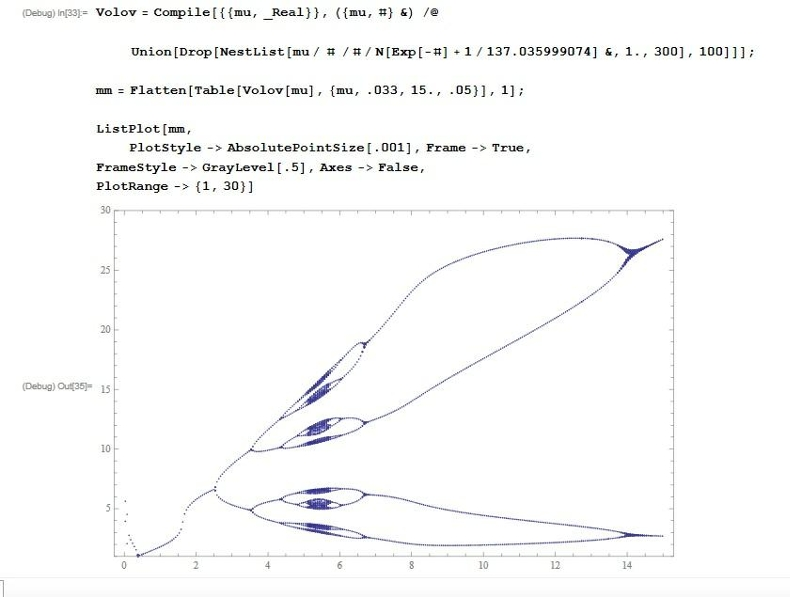The VRP-2 dynamics, with the parameter alpha value approaching the value equal to the value of the dimensionless ﬁne-structure constant, features maximum variety with regard to the number of bifurcations with the minimum degree of chaosticity.

D. B. Volov Specific behavior of one chaotic dynamics near the fine-structure constant http://arxiv.org/abs/1205.6091

http://chaosandcorrelation.org/Chaos/DV_1_5_2012.pdf

Volov_D__VTP_5_2011.pdf

http://www.sciteclibrary.ru/rus/catalog/pages/11612.html

This files contains an articles describing the Verhulst-Ricker-Planck dynamic and its relation to the fine structure constant.

The MathCAD's text program for the bifurcation diagram "four rats" (D.B.Volov, Russia, Samara).Source code in MATLAB to reproduce the bifurcation diagram "four rats(A.P.Trounev, Toronto, Canada)

(smile must be replaced by a colon)

x(i+1)=-L(k)/(x(i)^2(exp(x(i))+alpha))

L=zeros(1,500);

y=zeros(1,128);
ly=zeros(1,128);
alpha=1/137.035999074;
dL=(3.7+exp(1.1989))/500;

for k=1:length(L)

for i=1:length(y)

if (k==1)

L(k)=-3.8;

y(k,=1;
ly(k,=log(y(k,);

else

if (i==1)

L(k)=L(k-1)+dL;
V=y(k-1,128)*y(k-1,128)*(exp(-y(k-1,128))+alpha);

y(k,i)=exp(L(k))/V;
ly(k,i)=log(y(k,i));

else
V1=y(k,i-1)*y(k,i-1)*(exp(-y(k,i-1))+alpha);
y(k,i)=exp(L(k))/V1;
ly(k,i)=log(y(k,i));

end

end

end
end

for i=1:128

plot(L,ly(:,i),'.k')

hold on

title('a=1/137');

xlabel('lnK');ylabel('lnx');

end

The result:The bifurcation diagram "four ratsone-dimensional dynamic.Code for the Wolfram Mathematica 8 & result (A.P.Trounev, Toronto, Canada):# Nonlinear Phenomena.

#### 1. Elsevier

Physica D (Nonlinear Phenomena)

Physics Letters A

Communications in Nonlinear Science and Numerical Simulation

International Journal of Non-Linear Mechanics

Fluid Dynamics Research

Chaos, Solitons & Fractals